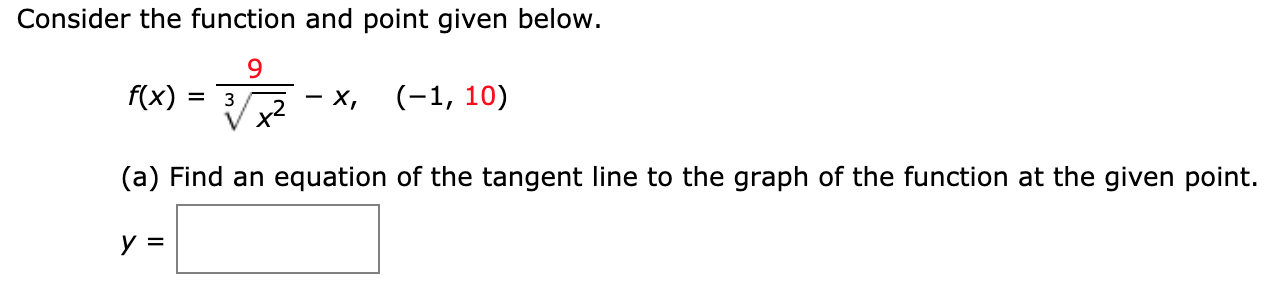# Consider the function and point given below.f(x)(-1, 10)х,х*(a) Find an equation of the tangent line to the graph of the function at the given point.У 3

Question
3 viewshelp_outlineImage TranscriptioncloseConsider the function and point given below. f(x) (-1, 10) х, х* (a) Find an equation of the tangent line to the graph of the function at the given point. У 3 fullscreen
check_circle

Step 1

Consider the given function:

Step 2

To find the equation of a tangent line first find its derivative...help_outlineImage Transcriptionclose9 f(x) 9 f(x) ro- -18 S(e)=9 2 f(x)9 3 |-1= -1= 1 3.r3 -6 -6 -1=6-1 =5 -1 f() (-)3 fullscreen

### Want to see the full answer?

See Solution

#### Want to see this answer and more?

Solutions are written by subject experts who are available 24/7. Questions are typically answered within 1 hour.*

See Solution
*Response times may vary by subject and question.
Tagged in

### Calculus